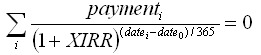## nyholm/effective-interest-rate

A library to calculate effective interest rate. Also know as XIRR or effective APR.

##### Details

Homepage

Source

Issues

12 119

14

1

3

1.0.0 2017-04-04 14:45 UTC

This is a library that calculates the effective interest rate. The effective interest could also be called XIRR or effective APR. This is the PHP library. You will find a JavaScript version of this library here.

## Examples

### Equal payments

If you are do a car loan of 100 000 Money. The loan is for 48 months and you pay 2 400 Money every month. What is the effective interest?

We guess that it is somewhere around 3%.

```use Nyholm\EffectiveInterest\Calculator;

\$principal = 100000;
\$payment = 2400;
\$numberOfMonths = 48;
\$guess = 0.03;
\$calculator = new Calculator();

\$interest = \$calculator->withEqualPayments(\$principal, \$payment, \$numberOfMonths, \$guess);

echo \$interest; // 0.07115```

Correct answer is 7.12%

### Specified payments

What if the payments are not equal? The first payment has an administration fee of 400 Money and we like to pay the rest of the loan after 36 months. So the 36th payment will be 31 200 Money.

```use Nyholm\EffectiveInterest\Calculator;

\$principal = 100000;
\$payment = 2400;
\$guess = 0.03;
\$startDate = '2017-04-30';
\$calculator = new Calculator();

\$payments = [
'2017-04-30' => \$payment + 400,
'2017-05-31' => \$payment,
'2017-06-30' => \$payment,
'2017-07-31' => \$payment,
// More dates
'2019-12-31' => \$payment,
'2020-01-31' => \$payment,
'2020-02-28' => \$payment,
'2020-03-31' => 31200,
];

\$interest = \$calculator->withSpecifiedPayments(\$principal, \$startDate, \$payments, \$guess);

echo \$interest; // 0.084870```

Correct answer is 8.49%

## The mathematics

We are using the same formula that Excel's XIRR function is using. We are also using NewtonRaphsons method to numerically find the interest we are looking for.